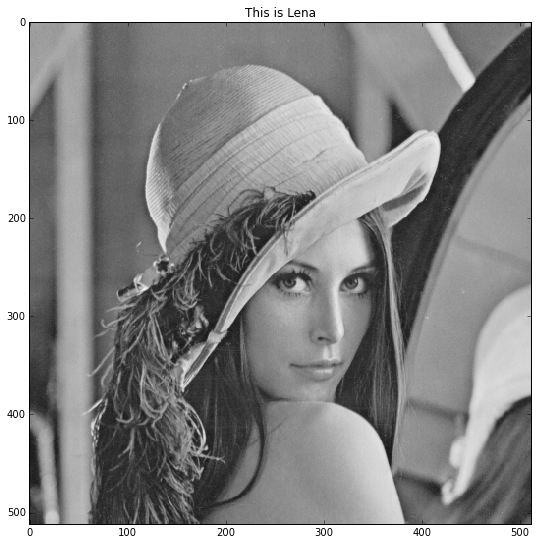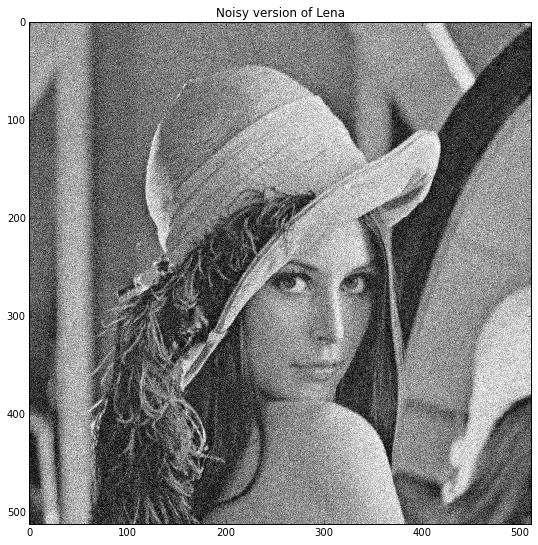# Forward-Backward method on the dual problem¶

This numerical tour is an introduction to convex duality with an application to total variation denoising.

$\newcommand{\dotp}{\langle #1, #2 \rangle}$ $\newcommand{\enscond}{\lbrace #1, #2 \rbrace}$ $\newcommand{\pd}{ \frac{ \partial #1}{\partial #2} }$ $\newcommand{\umin}{\underset{#1}{\min}\;}$ $\newcommand{\umax}{\underset{#1}{\max}\;}$ $\newcommand{\umin}{\underset{#1}{\min}\;}$ $\newcommand{\uargmin}{\underset{#1}{argmin}\;}$ $\newcommand{\norm}{\|#1\|}$ $\newcommand{\abs}{\left|#1\right|}$ $\newcommand{\choice}{ \left\{ \begin{array}{l} #1 \end{array} \right. }$ $\newcommand{\pa}{\left(#1\right)}$ $\newcommand{\diag}{{diag}\left( #1 \right)}$ $\newcommand{\qandq}{\quad\text{and}\quad}$ $\newcommand{\qwhereq}{\quad\text{where}\quad}$ $\newcommand{\qifq}{ \quad \text{if} \quad }$ $\newcommand{\qarrq}{ \quad \Longrightarrow \quad }$ $\newcommand{\ZZ}{\mathbb{Z}}$ $\newcommand{\CC}{\mathbb{C}}$ $\newcommand{\RR}{\mathbb{R}}$ $\newcommand{\EE}{\mathbb{E}}$ $\newcommand{\Zz}{\mathcal{Z}}$ $\newcommand{\Ww}{\mathcal{W}}$ $\newcommand{\Vv}{\mathcal{V}}$ $\newcommand{\Nn}{\mathcal{N}}$ $\newcommand{\NN}{\mathcal{N}}$ $\newcommand{\Hh}{\mathcal{H}}$ $\newcommand{\Bb}{\mathcal{B}}$ $\newcommand{\Ee}{\mathcal{E}}$ $\newcommand{\Cc}{\mathcal{C}}$ $\newcommand{\Gg}{\mathcal{G}}$ $\newcommand{\Ss}{\mathcal{S}}$ $\newcommand{\Pp}{\mathcal{P}}$ $\newcommand{\Ff}{\mathcal{F}}$ $\newcommand{\Xx}{\mathcal{X}}$ $\newcommand{\Mm}{\mathcal{M}}$ $\newcommand{\Ii}{\mathcal{I}}$ $\newcommand{\Dd}{\mathcal{D}}$ $\newcommand{\Ll}{\mathcal{L}}$ $\newcommand{\Tt}{\mathcal{T}}$ $\newcommand{\si}{\sigma}$ $\newcommand{\al}{\alpha}$ $\newcommand{\la}{\lambda}$ $\newcommand{\ga}{\gamma}$ $\newcommand{\Ga}{\Gamma}$ $\newcommand{\La}{\Lambda}$ $\newcommand{\si}{\sigma}$ $\newcommand{\Si}{\Sigma}$ $\newcommand{\be}{\beta}$ $\newcommand{\de}{\delta}$ $\newcommand{\De}{\Delta}$ $\newcommand{\phi}{\varphi}$ $\newcommand{\th}{\theta}$ $\newcommand{\om}{\omega}$ $\newcommand{\Om}{\Omega}$

In :
from __future__ import division
%pylab inline

Populating the interactive namespace from numpy and matplotlib


## Convex Duality¶

Given a convex, proper, and lower semi-continuous function $f$ defined on $\RR^N$, its conjugate $f^*$ is the convex function defined as $$\forall u \in \RR^N, \quad f^*(u) = \umax{x \in \RR^N} \dotp{x}{u} - f(x).$$

One has $(f^*)^*=f$.

One can show that if $f(x) = \frac{1}{2} \norm{A x - b}^2$, where $A \in \RR^{N \times N}$ is an invertible matrix, then $$f^*(u) = \frac{1}{2} \norm{(A^*)^{-1} u + b}^2- \frac{1}{2}\norm{b}^2$$

One can show that in the case of $\ell^p$ norms $$f(x) = \norm{x}_p = \pa{ \sum_{i=1}^N \abs{x_i}^p }^{1/p},$$ with the usual extension to $p=+\infty$ $$\norm{x}_\infty = \umax{1 \leq i \leq N} \abs{x_i},$$ then one has $$f^* = \iota_{\norm{\cdot}_q \leq 1} \qwhereq \frac{1}{p}+\frac{1}{q}=1,$$ where $\iota_{\Cc}$ is the indicator function of the convex set $\Cc$.

## Forward-backward on the Dual Problem¶

We are concerned with the minimization of composite problems of the form $$\umin{x \in \RR^N} f(x) + g(Lx)$$ where $L \in \RR^{P \times N}$ is a linear operator (a matrix), $f : \RR^N \rightarrow \RR$ and $g : \RR^P \rightarrow \RR$ are convex functionals.

We now assume that $f$ is $\beta$-strongly convex. Then, $f^*$ is differentiable and its gradient is $\beta$-Lipschitz continuous.

In this case, the Fenchel-Rockafellar theorem shows that one can solve the following dual problem $$\umin{u \in \RR^P} f^*( -L^* u ) + g^*(u)$$ and recover the unique solution $x^\star$ of the primal problem from a (non-necessarily unique) solution $u^\star$ of the dual problem, as $$x^\star = \nabla f^*( -L^* u^\star ).$$

Moreover, the optimal primal and dual costs satisfy $$E^\star= f(x^\star) + g(Lx^\star) = • f^( -L^ u^\star ) - g^*(u^\star).$$

Denoting $F(u) = f^*( -L^* u )$ and $G(u) = g^*(u)$, one thus needs to solve the problem $$\umin{u \in \RR^P} F(u) + G(u).$$

We assume that the function $g$ is simple, in the sense that one can apply the proximity operator $\mathrm{prox}_{\ga g}$ for any $\ga > 0$.

Then, $G=g^*$ is simple as well, because of the Moreau identity $$x = \text{prox}_{\ga g^*}(x) + \ga \text{prox}_{g/\ga}(x/\ga).$$

Since $F$ is smooth and $G$ is simple, one can apply the forward-backward algorithm, which reads, after initilizing $u^{(0)} \in \RR^P$, $$u^{(k+1)} = \text{prox}_{\ga G}\pa{ u^{(k)} - \ga \nabla F( u^{(k)} ) }.$$

The primal iterates are defined as $$x^{(k)} = \nabla f^*( -L^* u^{(k)} ).$$

So, we can write the algorithm in the primal-dual form $$\left|\begin{array}{l} x^{(k)} = \nabla f^*( -L^* u^{(k)} )\\ u^{(k+1)} = \text{prox}_{\ga g^*}\pa{ u^{(k)} + \ga Lx^{(k)}}. \end{array}\right.$$

If $0< \ga < 2/(\beta\|L\|^2)$, $x^{(k)}$ converges to a primal solution $x^\star$ and $u^{(k)}$ converges to a dual solution $u^\star$.

## Total Variation¶

Like in lab 1, we are interested in imaging inverse problems: we want to estimate the unknown sharp image $s^\sharp$ from the data $y=Ax^\sharp\ +\mbox{ noise}$, where $A$ is the linear observation operator.

In lab 1, we defined the 'discrete gradient' $D$ and we used as regularizer the Tikhonov functional $\frac{1}{2}\|Dx\|^2_{2,2}=\frac{1}{2}\sum_{n_1,n_2} \|(Dx)_{n_1,n_2}\|^2_2=\frac{1}{2}\sum_{n_1,n_2} (Dx)_{n_1,n_2,v}^2+(Dx)_{n_1,n_2,h}^2$.

A better regularizer for imaging problems is the total variation, which corresponds to taking the $\ell_1$ norm instead of the squared $\ell_2$ norm of the discrete gradient:

$$\mathrm{TV}(x)=\|Dx\|_{1,2}=\sum_{n_1,n_2} \|(Dx)_{n_1,n_2}\|_2=\sum_{n_1,n_2} \sqrt{ (Dx)_{n_1,n_2,v}^2+(Dx)_{n_1,n_2,h}^2}.$$

The total variation was first introduced in L. I. Rudin, S. Osher, E. Fatemi, "Nonlinear total variation based noise removal algorithms," Physica D, vol. 60, 1992.

The difficulty is that there is no way to compute the proximity operator of the total variation in 2-D (fast exact algorithms exist in 1-D). So, we must view it as the simple functional $\lambda \|\cdot\|_{1,2}$ composed with the linear operator $D$. Indeed, the proximity operator of $\lambda \|\cdot\|_{1,2}$ is simply elementwise soft-thresholding of the magnitude of the vector field.

So, if $A$ is invertible, the dual forward-backward algorithm is appropriate to solve least-squares problems regularized with the total-variation.

## Denoising Lena¶

In the following, we consider the image denoising problem, i.e. $A=\mathrm{Id}$, applied to the Lena image. So, given the noisy image $y$, we want to estimated the clean Lena image $x^\sharp$ by the image $x^\star$ solution to the strongly convex primal problem

$$\umin{x \in \RR^{N_1\times N_2}} E_p(x)= f(x)+g(Dx)=\frac{1}{2}\|x-y\|^2+\lambda\|Dx\|_{1,2}$$

The dual problem is

$$\umin{u \in \RR^{N_1\times N_2\times 2}} E_d(u)= f^*(-D^*u)+g^*(u)=\frac{1}{2}\|-D^*u+y\|^2- \frac{1}{2}\norm{y}^2+\imath_{\|\cdot\|_{\infty,2}\leq\lambda}(u)$$

## Chambolle Dual Algorithm ¶

We will solve the total-variation regularized denoising problem by applying the forward-backward algorithm on the dual problem. This is sometimes called the Chambolle Dual Algorithm, because this idea was initially proposed in A. Chambolle, "An Algorithm for Total Variation Minimization and Applications", Journal of Mathematical Imaging and Vision, 20(1-2), 2004.

Note that since $g^*$ is an indicator function, the forward-backward algorithm reverts to projected gradient descent.

For a more general framework, see P. L. Combettes, D. Dung, and B. C. Vu, "Dualization of signal recovery problems", Set-Valued and Variational Analysis, vol. 18, 2010

We create the noisy image $y$.

In :
from scipy import misc
xsharp = misc.lena()
xsharp = xsharp.astype(float32)

In :
figsize(9,9)
imshow(xsharp, interpolation='nearest', cmap=cm.gray, vmin=0, vmax=255)
title('This is Lena')

Out:
<matplotlib.text.Text at 0x108dc94d0>In :
(N1,N2) = shape(xsharp)
noiselevel = 20
y = xsharp + noiselevel * randn(N1,N2)

In :
figsize(9,9)
imshow(y, interpolation='nearest', cmap=cm.gray, vmin=0, vmax=255)
title('Noisy version of Lena')

Out:
<matplotlib.text.Text at 0x10a488d90>We define the discrete gradient operator $D$ and its adjoint $D^*$.

In :
D = lambda x : c_['2,3',r_[diff(x,1,0), zeros([1,x.shape])],c_[diff(x,1,1), zeros([x.shape,1])]]

In :
Dadj = lambda v : r_['0,2',-v[0,:,0],-diff(v[:-1,:,0],1,0),v[-2,:,0]] + c_['1,2',-v[:,0,1],-diff(v[:,:-1,1],1,1),v[:,-2,1]]


The gradient of $f^*$ is simply $\nabla f^*:x\mapsto x+y$.

In :
def grad_f_conj (x,y) :
return x + y


The proximity operator $\text{prox}_{\ga g^*}$ does not depend on $\gamma$ and is such that $$\big(\text{prox}_{\ga g^*}(u)\big)_{n_1,n_2}=\frac{1}{\max(\|u_{n_1,n_2}\|_2/\lambda,1)}u_{n_1,n_2}\in\mathbb{R}^2,\quad\forall (n_1,n_2).$$

In :
def prox_g_conj (u, Lambda) :
return u/tile(maximum(sqrt(sum(u**2,2,keepdims=True))/Lambda,1),(1,1,2)) # soft-thresholding


### __Exercise: write the code of the dual forward-backward iteration for denoising.__

In :
Lambda = 25
gamma = 1.9/8 # we must have 0 < gamma < 2/8
nbiter = 400
(N1,N2) = y.shape
u = zeros([N1,N2,2])
Ep_array = zeros(nbiter) # array for the primal energy E_p
Ed_array = zeros(nbiter) # array for the dual energy E_d
sqnormy = norm(y)**2/2
for iter in range(nbiter):
Ep_array[iter] = norm(x-y)**2/2 + Lambda*sum(sqrt(sum(D(x)**2,2)))

figsize(9,9)

<matplotlib.text.Text at 0x108bd3610>